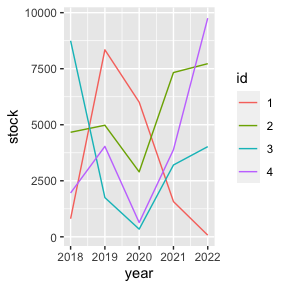# Column formats

library(tibble)

## Overview

This vignette shows how to decorate columns for custom formatting. We use the formattable package for demonstration because it already contains useful vector classes that apply a custom formatting to numbers.

library(formattable)

tbl <- tibble(x = digits(9:11, 3))
tbl
#> # A tibble: 3 × 1
#>   x
#>   <formttbl>
#> 1 9.000
#> 2 10.000
#> 3 11.000

The x column in the tibble above is a regular number with a formatting method. It always will be shown with three digits after the decimal point. This also applies to columns derived from x.

library(dplyr)
tbl2 <-
tbl %>%
mutate(
y = x + 1,
z = x * x,
v = y + z,
lag = lag(x, default = x[]),
sin = sin(x),
mean = mean(v),
var = var(x)
)

tbl2
#> # A tibble: 3 × 8
#>           x         y         z         v       lag       sin      mean   var
#>   <dbl:fmt> <dbl:fmt> <dbl:fmt> <dbl:fmt> <dbl:fmt> <dbl:fmt> <dbl:fmt> <dbl>
#> 1     9.000    10.000    81.000    91.000     9.000     0.412   111.667     1
#> 2    10.000    11.000   100.000   111.000     9.000    -0.544   111.667     1
#> 3    11.000    12.000   121.000   133.000    10.000    -1.000   111.667     1

Summaries also maintain the formatting.

tbl2 %>%
group_by(lag) %>%
summarize(z = mean(z)) %>%
ungroup()
#> # A tibble: 2 × 2
#>         lag         z
#>   <dbl:fmt> <dbl:fmt>
#> 1     9.000    90.500
#> 2    10.000   121.000

Same for pivoting operations.

library(tidyr)

stocks <-
expand_grid(id = factor(1:4), year = 2018:2022) %>%
mutate(stock = currency(runif(20) * 10000))

stocks %>%
pivot_wider(id_cols = id, names_from = year, values_from = stock)
#> # A tibble: 4 × 6
#>   id       2018    2019    2020    2021    2022
#>   <fct> <dbl:fmt> <dbl:fmt> <dbl:fmt> <dbl:fmt> <dbl:fmt>
#> 1 1       $807.50$8,343.33 $6,007.61$1,572.08    $73.99 #> 2 2$4,663.93 $4,977.77$2,897.67 $7,328.82$7,725.22
#> 3 3     $8,746.01$1,749.41   $342.41$3,203.86 $4,023.28 #> 4 4$1,956.70 $4,035.38$636.61 $3,887.01$9,755.48

For ggplot2 we need to do some work to show apply the formatting to the scales.

library(ggplot2)

# Needs https://github.com/tidyverse/ggplot2/pull/4065 or similar
stocks %>%
ggplot(aes(x = year, y = stock, color = id)) +
geom_line()It pays off to specify formatting very early in the process. The diagram below shows the principal stages of data analysis and exploration from “R for data science”.

The subsequent diagram adds data formats, communication options, and explicit data formatting. The original r4ds transitions are highlighted in bold. There are two principal options where to apply formatting for results: right before communicating them, or right after importing.

Applying formatting early in the process gives the added benefit of showing the data in a useful format during the “Tidy”, “Transform”, and “Visualize” stages. For this to be useful, we need to ensure that the formatting options applied early:

• give a good user experience for analysis
• are easy to set up
• keep sticky in the process of data analysis and exploration
• support the analyst in asking the right questions about the data
• convey the critical information at a glance, with support to go into greater detail easier
• look good for communication
• are applied in the various communication options
• support everything necessary to present the data in the desired way

Ensuring stickiness is difficult, and is insufficient for a dbplyr workflow where parts of the “Tidy”, “Transform” or even “Visualize” stages are run on the database. Often it’s possible to derive a rule-based approach for formatting.

tbl3 <-
tibble(id = letters[1:3], x = 9:11) %>%
mutate(
y = x + 1,
z = x * x,
v = y + z,
lag = lag(x, default = x[]),
sin = sin(x),
mean = mean(v),
var = var(x)
)

tbl3
#> # A tibble: 3 × 9
#>   id        x     y     z     v   lag        sin     mean   var
#>   <chr> <int> <dbl> <int> <dbl> <int>      <dbl>    <dbl> <dbl>
#> 1 a         9    10    81    91     9  0.4121185 111.6667     1
#> 2 b        10    11   100   111     9 -0.5440211 111.6667     1
#> 3 c        11    12   121   133    10 -0.9999902 111.6667     1

tbl3 %>%
mutate(
across(where(is.numeric), ~ digits(.x, 3)),
across(where(~ is.numeric(.x) && mean(.x) > 50), ~ digits(.x, 1))
)
#> # A tibble: 3 × 9
#>   id            x         y         z         v       lag       sin   mean   var
#>   <chr> <dbl:fmt> <dbl:fmt> <dbl:fmt> <dbl:fmt> <dbl:fmt> <dbl:fmt> <dbl:> <dbl>
#> 1 a         9.000    10.000      81.0      91.0     9.000     0.412  111.7 1.000
#> 2 b        10.000    11.000     100.0     111.0     9.000    -0.544  111.7 1.000
#> 3 c        11.000    12.000     121.0     133.0    10.000    -1.000  111.7 1.000

These rules can be stored in quos():

rules <- quos(
across(where(is.numeric), ~ digits(.x, 3)),
across(where(~ is.numeric(.x) && mean(.x) > 50), ~ digits(.x, 1))
)

tbl3 %>%
mutate(!!!rules)
#> # A tibble: 3 × 9
#>   id            x         y         z         v       lag       sin   mean   var
#>   <chr> <dbl:fmt> <dbl:fmt> <dbl:fmt> <dbl:fmt> <dbl:fmt> <dbl:fmt> <dbl:> <dbl>
#> 1 a         9.000    10.000      81.0      91.0     9.000     0.412  111.7 1.000
#> 2 b        10.000    11.000     100.0     111.0     9.000    -0.544  111.7 1.000
#> 3 c        11.000    12.000     121.0     133.0    10.000    -1.000  111.7 1.000

This poses a few drawbacks:

• The syntax is repetitive and not very intuitive
• Rules that match multiple columns must be given in reverse order due to the way mutate() works, and are executed multiple times

What would a good API for rule-based formatting look like?